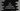# C# program to count the total number of vowels in a string## How to count the total number of vowels in a string in C#:

In this post, we will learn how to count the total number of vowels in a user input string in C#. Our program will read the string as input from the user, calculate the total number of vowels in that string and print that value to the user.

The program will use a for loop to iterate through the characters of the string. For each character, it will check if it is a vowel or not. If it is a vowel, it will increment the valud of a counter that is initialized as 0 at the start of the program. Once the program will end, the counter variable will hold the total number of vowels. At the end of the program, the program will print this variable, i.e. total number of vowels.

### C# program:

Below is the complete C# program:

``````using System;

namespace HelloWorld
{
public class Program
{
public static void Main(string[] args)
{
String givenString = "Hello WOrld !!";
String vowels = "aeiouAEIOU";
int total = 0;

for (int i = 0; i < givenString.Length; i++)
{
if (vowels.Contains(givenString[i]+""))
{
total++;
}
}

Console.WriteLine("Total vowels: " + total);
}
}
}``````

Here,

• givenString is the given string.
• vowels is a string with all the vowels. It includes all the characters including lower-case and upper-case vowels.
• total variable is used to hold the total number of vowels.
• The for loop runs from i = 0 to length of the string givenString.
• For each character in the string, it is checking if that character is in the vowels string or not. We are using Contains method to check if the character is in vowels. But, Contains takes one string. So, we are appending an empty string with the character to change it to a string.
• If the character is in vowels, increment the value of total by 1.
• Finally, print the value of total.

If you run the above program, it will print the below output:

``Total vowels: 3``